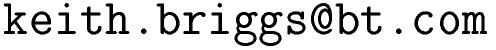Keith Briggshome · papers · thesis · talks · meetings · records · maths notes · software « · languages · music · travel · cv · students · maps · place-names · people · photos · links · ex libris · site map### Ode

#### Overview

This is a Unix command-line ordinary differential equation solver. It is intended for use as a filter in shell pipelines, in the spirit of statistics and data-processing codes like TISEAN and |stat.

There are two interfaces:

1. the C program interface Ode uses a very abbreviated syntax where dependent variables (20 maximum) must be named a,b,c,...,w. The independent variable is always named x, and the order of arguments is significant. The expressions give the right-hand sides of the ODEs; the left-hand side is implicit. Thus, for example, just "a" stands for "da/dx=a", while "b -1 sin(x)" stands for "da/dx=b; db/dx=-1; dc/dx=sin(x)".

2. the friendly python interface ode.py which accepts meaningful dependent variable names (20 maximum), the independent variable is anonymous (but could be named t, for example, by including the equations t.=1 t=0), and the order of arguments is not significant.

The output is a table of values suitable for piping to a plotting utility (for example, graph -TX -C).

Ode was designed as a friendlier and more robust version of ode in GNU plotutils (hence the O to distinguish it). It also aims for the highest possible accuracy.

Keith Briggs

#### How does it work?

It uses the formulc expression parser by Helfgott and a slightly modified version of the eighth-order Runge-Kutta code dop853.c by Hairer. (All required source files are included in the Ode package.) Special compensated summation tricks are used to enable high accuracy by reducing roundoff error effects. I also make use of the continuous interpolation facility of Hairer's code to produce the output table. The python interface ode.py just translates its input arguments and then calls Ode; thus, there is no loss of efficiency.

Ode.tgz

#### Installation

1. tar zvxf Ode.tgz
2. cd Ode
3. Edit the top lines of makefile to choose your C compiler and set the X86 option for Intel x86 processors (including Pentium).
4. Edit the top line of ode.py to point to your python interpreter.
5. Edit ./Ode in ode.py to point to your executable of Ode.
6. make

#### Usage

The dumb interface (for experts, or those without python installed):

Ode [-reps1] [-aeps2] rhs1 rhs2 ... iv1 iv2 ... t_start t_end number_of_steps

Here eps1 and eps2 are relative and absolute error tolerances, rhsx are righthand-side expressions (so that rhs1 implicitly means a'=rhs1) and ivx are initial value constants. The order of arguments must be strictly as above. The number of differential equations is (number of arguments-3)/2 (not counting the optional -r -a arguments).

The friendly interface (recommended):

ode.py var1.=rhs1 var2.=rhs2 ... var1=iv1 var2=iv2 ... [t_start:[number_of_steps]:t_end] [plotvar1,plotvar2,...]
(var'=rhs is also accepted for an ode specification)

Initial values can be python expressions which must evaluate to a Float constant. Default plotvars: all (including the independent variable as column 1); default t_start=0, t_end=1, number_of_steps=100.

Note that equations containing parentheses or using the '= notation must be quoted (using ") to protect them from the shell. Arguments may be in any order. The special syntax for the plot variable list 0,pv1,pv2,... means that the independent variable should NOT be output.

#### Examples

Some of these examples make use of a column extraction utility and a graphing utility.

Exponential growth:
Ode a 2 0 1 100
or
ode.py y.=2*y y=1
thus, to compute e:
Ode a 1 0 1 1

Logistic growth:
Ode "a*(1-a)" 0.1 0 5 100

Richards growth:
Ode "1.5*a*(1-a^2/16)" 0.1 0 4 100

How to integrate backwards from 1 to 0: compute LambertW0(1):
Ode "a/(1+a)" 1 1 0 1

Wright w function (iv is LambertW0(1)):
Ode "a/(1+a)" 0.5671432904097838730 0 10 100

Sine:
Ode b -a 0 1 0 6.3 100
or
ode.py sin.=cos cos.=-sin sin=0 cos=1 sin 0:100:6.3

Damped sine:
ode.py x.=y-x/5 y.=-x x=0 y=1 x 0:100:100

Pendulum:
ode.py theta.=omega "omega.=-sin(theta)" theta=0 omega=2 theta 0::100

Atwood machine:

Airy:
ode.py x.=1 y.=z z.=-x*y x=0 y=1 z=-1 y 0::15

Lotka-Volterra:
ode.py "hares'=hares-1.3*hares*foxes" "foxes'=1.5*hares*foxes-2*foxes" hares=1 foxes=2 0::20 hares

Duffing:
ode.py x=1 v=0 t=0 x.=v/6.28 "v.=(-x^3+x-v/4+0.3*cos(t))/6.28" t.=1 0::100 x

Lorenz:
ode.py x.=3*y-3*x y.=-x*z+26*x-y z.=x*y-z x=0 y=1 z=10 0,x,y 0:1000:30 | graph -TX -C

Roessler:
ode.py x.=-y-z y.=x+0.2*y z.=0.2+x*z-4*z x=1 y=0 z=0 0:200:200 x

Geisel's 2d diffusive chaotic system (Phys. Rev. Lett. 59, 2503 (1988)):
ode.py "x'=vx" "y'=vy" "vx'=(1.5+0.5*cos(y))*sin(x)" "vy'=(1.5+0.5*cos(x))*sin(y)" x=0 y=0 vx=1 vy=0.5 0,x,y 0:500:200 | graph -TX -C

Example of how to solve a non-autonomous system:
ode.py "y.= cos(3.14159*t*y)" y=1 t.=1 t=0

Planet around binary star system:
ode.py x.=vx y.=vy "vx.=-x/cub(sqrt(sqr(x)+sqr(y)))-(x+5)/cub(sqrt(sqr(x+5)+sqr(y)))" "vy.=-y/cub(sqrt(sqr(x)+sqr(y)))-y/cub(sqrt(sqr(x+5)+sqr(y)))" x=1 y=0 vx=0 vy=1.1 x 0:1000:50
or, using shell variables to simplify expressions:
denom1="cub(sqrt(sqr(x)+sqr(y)))"; denom2="cub(sqrt(sqr(x+5)+sqr(y)))"; ode.py x.=vx y.=vy "vx.=-x/\$denom1-(x+5)/\$denom2" "vy.=-y/\$denom1-y/\$denom2" x=1 y=0 vx=0 vy=1.15 0,x,y 0:1000:50 | graph -TX -C

Using shell variables to set a parameter:
rate=0.021; ode.py co2.=-\$rate*co2 co2=1

Using backticks to set a parameter from output of another program:
ode.py co2.=-`echo 0.021`*co2 co2=1

Setting initial values from output of another program through a pipe:
echo position=1 velocity=2 | xargs ode.py position.=velocity velocity.=-1/position^2 position 0::100

Bead sliding on a smooth circular wire:
ode.py "theta'= vtheta" "vtheta'=100*sin(theta)*cos(theta)-10*sin(theta)" theta=0.1 vtheta=0 theta 0::5

Chemical kinetics with four species (a+2b<=>c->d, all rates=1):
Ode c-a*b^2 c-a*b^2 a*b^2-c-c c 0.1 1 0 0 0 10 100

Example of a more complicated ode:
Ode "-0.05*sin(2*pi()*x/18.2)-0.06*sin(2*pi()*x/19)-0.05*sin(2*pi()*x/23)+0.6*a-4*a^3" 0.4 40 56 100

This website uses no cookies. This page was last modified 2013-05-12 10:17 by.